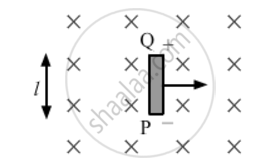# A Rod of Length L is Moved Horizontally with a Uniform Velocity 'V' in a Direction Perpendicular to Its Length Through a Region in Which a Uniform Magnetic Field is Acting Vertically Downward. Derive the Expression for the Emf Induced Across the Ends of the Rod. - Physics

A rod of length l is moved horizontally with a uniform velocity 'v' in a direction perpendicular to its length through a region in which a uniform magnetic field is acting vertically downward. Derive the expression for the emf induced across the ends of the rod.

#### SolutionConsider a rod PQ of length l moving in a magnetic field vecBwith a constant velocity vecv. The length of the rod is perpendicular to the magnetic field and the velocity is perpendicular to both field and the rod. The free electrons of the rod also move at this velocity v⃗  due to which it experiences a magnetic force

vec(F_b)=qvecvxxvecB

Here, q = 1.6×10-19 C is the charge of a electron. This force is towards Q to P. Hence, the free electrons will move towards P and positive charge appears at Q. An electrostatic field E is developed within the wire from Q to P. This field exerts a force

vec(F_b)=qvecE

on each free electron. The charge keeps on accumulating until

vec(F_b)=vec(F_e)

or

|qvecvxxvecB|=|qvecE|

⇒ vE

After this, there is no resultant force on the free electrons of the wire PQ. The potential difference between the ends Q and P is

EvBl

Thus, it is the magnetic force on the moving free electrons that maintains this potential difference and hence, produces an emf, e=Bvl

Concept: Magnetic Force
Is there an error in this question or solution?
2013-2014 (March) All India Set 3

Share